### IMO Shortlist 2005 problem C2

Kvaliteta:
Avg: 4,0
Težina:
Avg: 6,0
This ISL 2005 problem has not been used in any TST I know. A pity, since it is a nice problem, but in its shortlist formulation, it is absolutely incomprehensible. Here is a mathematical restatement of the problem:

Let$k$ be a nonnegative integer.

A forest consists of rooted (i. e. oriented) trees. Each vertex of the forest is either a leaf or has two successors. A vertex$v$ is called an extended successor of a vertex$u$ if there is a chain of vertices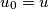$u_{0}=u$,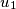$u_{1}$,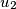$u_{2}$, ...,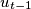$u_{t-1}$,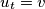$u_{t}=v$ with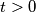$t>0$ such that the vertex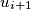$u_{i+1}$ is a successor of the vertex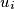$u_{i}$ for every integer$i$ with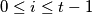$0\leq i\leq t-1$. A vertex is called dynastic if it has two successors and each of these successors has at least$k$ extended successors.

Prove that if the forest has$n$ vertices, then there are at most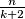$\frac{n}{k+2}$ dynastic vertices.
Izvor: Međunarodna matematička olimpijada, shortlist 2005